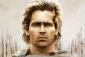# engineering economy

3 posts / 0 new
Sydney Salesengineering economy

A sum of Rs. 725 is lent in the beginning of a year at a certain rate of interest. After 8 months, a sum of Rs. 362.50 more is lent but at the rate twice the former. At the end of the year, Rs. 33.50 is earned as interest from both the loans. What was the interest earned in the principal of P725?
What is the interest earned in the principal of P362.50?

KMST

Since there is no mention of compounding or compounding term, we have to assume it is simple interest, and that makes the problem a simple algebra problem.
Let x = first loan interest rate, as a decimal (meaning that 4.0%=4.0/100=0.040, for example).
725x = interest (in Rs) earned on the first, year-long loan of Rs 725.
2x = interest rate for the second loan of Rs 362.50
(4/12)362.50(2x) = (1/3)725x = 725x/3 = interest (in Rs) earned during the last 12-8=4 months of the year on the second loan
So, the total interest earned (in Rs) is
725x + 725x/3 = (4/3)725x , and since that is Rs.33.50,
(4/3)725x = 33.50 --> 725x = 33.50(3/4) ---> 725x = 25.125
ANOTHER WAY:
The second loan is for half the principal but at twice the rate, so it would be the same amount of interest if the second loan were for the whole year.
As it is for 1/3 of a year, the interest for the second loan is 1/3 of the interest for the first loan.
So, if we define
X = interest for the first loan,
(1/3)X = interest for the second loan, and
X + (1/3)X = 33.50 ---> (4/3)X = 33.50 ---> X =(3/4)33.50 ---> X=25.125

AlexanderWhy the Rupee becomes Peso?

• Mathematics inside the configured delimiters is rendered by MathJax. The default math delimiters are $$...$$ and $...$ for displayed mathematics, and $...$ and $...$ for in-line mathematics.